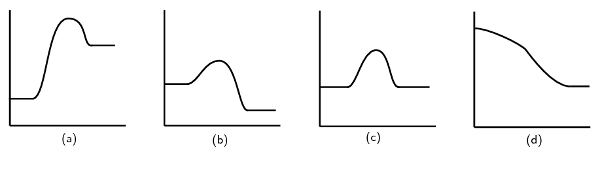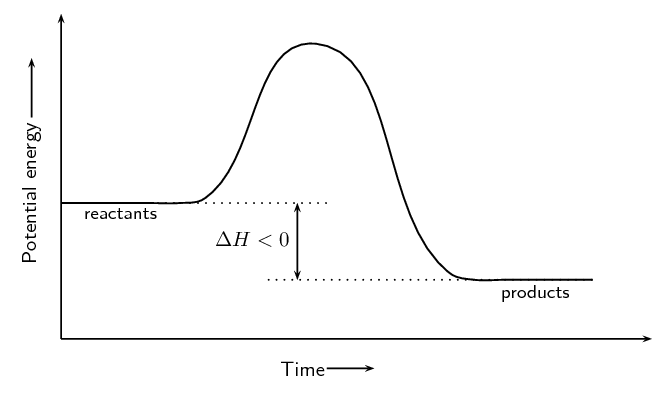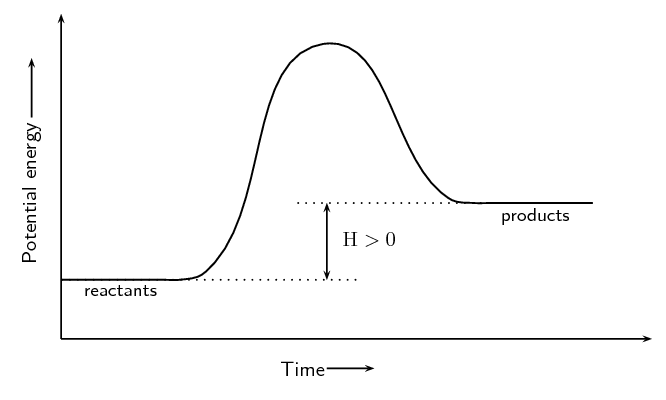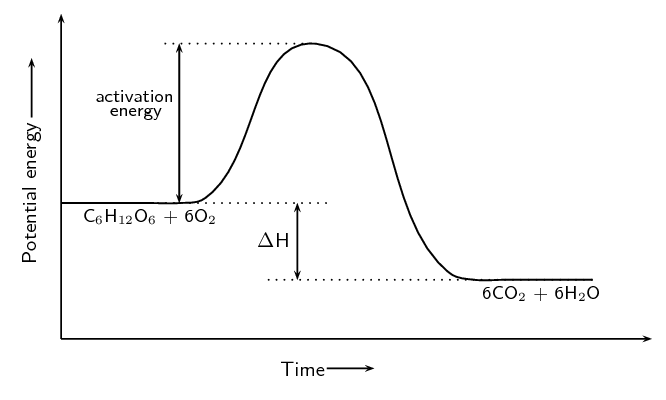Home Practice
For learners and parents For teachers and schools
Textbooks
Full catalogue
Pricing SupportLog in

We think you are located in United States. Is this correct?

# End of chapter exercises

Textbook Exercise 12.4

For each of the following, give one word or term for the description.

The minimum amount of energy that is needed for a reaction to proceed.

Activation energy

A measure of the bond strength in a chemical bond.

Bond energy

A type of reaction where ΔH is less than zero.

Exothermic reaction

A type of reaction that requires heat or light to proceed.

Endothermic reaction

For the following reaction:

$\text{HCl(aq)} + \text{NaOH(aq)} \rightarrow \text{NaCl(aq)} + \text{H}_{2}\text{O(ℓ)}$

choose the correct statement from the list below.

1. Energy is taken in when the new bonds in $$\text{NaCl}$$ are formed.

2. Energy is released when the bonds in $$\text{HCl}$$ break.

3. Energy is released when the bonds in $$\text{H}_{2}\text{O}$$ form.

4. Energy is released when the bonds in $$\text{NaOH}$$ break.

Energy is released when the bonds in $$\text{H}_{2}\text{O}$$ form.

For the following reaction:

$\text{A} + \text{B} → \text{AB} \qquad Δ\text{H} = -\text{129}\text{ kJ·mol^{-1}}$

choose the correct statement from the list below.

1. The energy of the reactants is less than the energy of the product.

2. The energy of the product is less than the energy of the reactants.

3. The reaction needs energy to occur.

4. The overall energy of the system increases during the reaction.

The energy of the product is less than the energy of the reactants. This is an exothermic reaction ($$\Delta \text{H} > 0$$).

Consider the following chemical reaction:

$2\text{NO}_{2}\text{(g)} \rightarrow \text{N}_{2}\text{O}_{4}\text{(g)} \qquad Δ\text{H} < \text{0}$

Which one of the following graphs best represents the changes in potential energy that take place during the production of $$\text{N}_{2}\text{O}_{4}$$?The second graph (b) is correct. The reaction is exothermic ($$\Delta \text{H} > 0$$), so the energy of the reactants must be greater than the energy of the products. Graphs (c) and (d) are not valid energy graphs.

In each of the following reactions, say whether the reaction is endothermic or exothermic, and give a reason for your answer. Draw the resulting energy graph for each reaction.

$$\text{Fe}_{2}\text{O}_{3}\text{(s)} + 2\text{Al(s)} → 2\text{Fe(s)} + \text{Al}_{2}\text{O}_{3} \text{(s)} + \text{ heat}$$

Exothermic. Heat is given off and this is represented by showing $$+$$ heat on the right hand side of the equation.$$\text{NH}_{4}\text{Cl(s)} + \text{heat} → \text{NH}_{3}\text{(g)} + \text{HCl(g)}$$

Endothermic. Energy is needed (shown by + energy on the left hand side of the reaction equation) for the reaction to proceed.The cellular respiration reaction is catalysed by enzymes. The equation for the reaction is:

$\text{C}_{6}\text{H}_{12}\text{O}_{6}\text{(s)} + 6\text{O}_{2}\text{(g)} → 6\text{CO}_{2}\text{(g)} + 6\text{H}_{2}\text{O(ℓ)}$

The change in potential energy during this reaction is shown below:Will the value of ΔH be positive or negative? Give a reason for your answer.

Negative. The energy of the products is less than the energy of the reactants.

Explain what is meant by activation energy.

The activation energy is the minimum energy that must be overcome to enable the reaction to proceed.

Glucose is one of the reactants in cellular respiration. What important chemical reaction produces glucose?

Photosynthesis